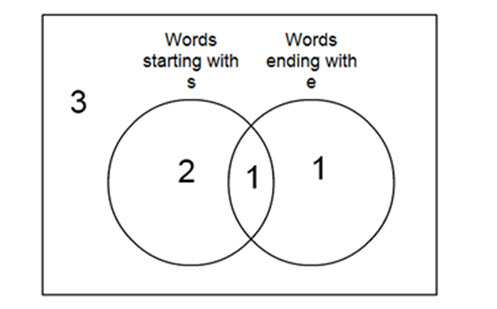Go to website

# Introducing Venn diagrams

This is the first in a series of Syllabus Bites related to Venn diagrams. Students classify data involving two attributes using Venn diagrams. They draw Venn diagrams and solve an open-ended problem by interpreting a Venn diagram.

Year level(s) Year 8
Audience Teacher
Format Interactive
Keywords Venn diagram, sorting, sets

## Curriculum alignment

Curriculum connections Critical and creative thinking
Strand and focus Probability, Apply understanding, Build understanding
Topics Chance and probability
AC: Mathematics (V9.0) content descriptions
AC9M8P02
Determine all possible combinations for 2 events, using two-way tables, tree diagrams and Venn diagrams, and use these to determine probabilities of specific outcomes in practical situations

Numeracy progression Understanding chance (P6)